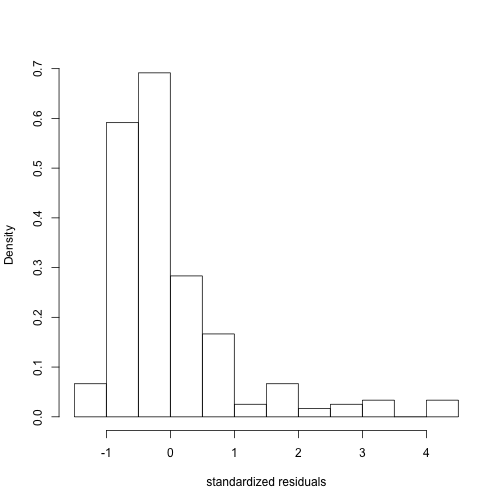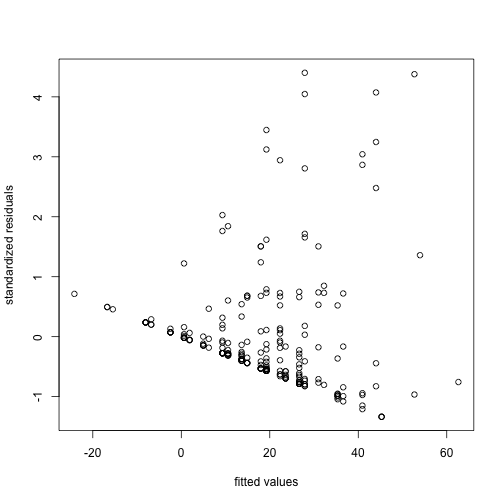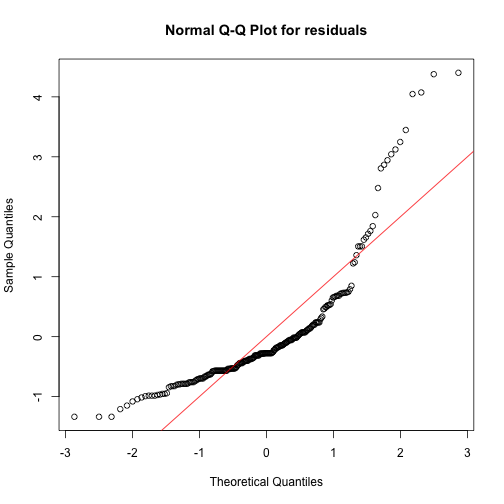# Abstract

The netregR package provides methods for performing regression of a network response, where each data point represents an edge on a network, on covariates of interest. In this vignette we first address linear regression of a single representation of a network. We provide examples for for directed and undirected networks, each with complete and incomplete observations. We then address linear regression for multiple observations of a network. Lastly, we examine some supporting methods for inverting covariance matrices of complete networks that are exchangeable. This vignette and the package netregR are based on @marrs2017.

# Introduction

We focus on the model \begin{align} y{ij} = {\bf{x}}{ij}T \boldsymbol{\beta} + \epsilon{ij}, \label{eq_linmod} \end{align} where $y{ij}$ is a (possibly directed) continuous measure from actor $$i$$ to actor $$j$$ and $${\bf{x}}_{ij}^T$$ is a corresponding vector of covariates. In our setting, the target is accurate inference of $$\boldsymbol{\beta}$$. As $$y_{ij}$$ is a network, we expect correlation among the errors $$\{ \epsilon_{ij} \}_{i,j}^n$$. In @marrs2017, we argue that it is reasonable to assume that the errors are jointly exchangeable. By this, we mean that the labeling of the actors is non-informative to the distirbution of $$\{ \epsilon_{ij} \}_{i,j}^n$$, such that the distribution $$\mathbb{P}(\{ \epsilon_{ij} \}_{i,j}^n) = \mathbb{P}(\{ \epsilon_{\pi(i) \pi(j)} \}_{i,j}^n)$$ for any permutation $$\pi(.)$$. Indeed, many network models for residual structure are jointly exchangeable, for example the bilinear mixed effects network regression model proposed in @hoff2005.

This vignette proceeds as follows: we first briefly described the regression methods implemented in the following section. Then, we provide an example of ordinary least squares and weighted least squares regressions of real world network data. We use a synthetic data set to illustrate the use of our methods on three-dimensional relational data, where the relations among the same set of actors has multiple observations over different contexts. Finally, we provide examples of efficient inversion of covariance matrices of jointly exchangeable relational data (in the single observation case).

### Ordinary Least Squares (OLS)

We may estimate the linear model using ordinary least squares $$\widehat{\boldsymbol{\beta}}_{OLS} = (X^TX)^{-1}X^TY$$, where $$X$$ is a matrix consisting of rows made up of $${\bf{x}}_{ij}^T$$ and $$Y$$ is a vector of the corresponding network responses. However, for accurate inference of $$\widehat{\boldsymbol{\beta}}_{OLS}$$, we must estimate \begin{align} {V}\left[\widehat{\boldsymbol{\beta}}{OLS} \ \big| \ X \right] = (XTX){-1}XT {V}[\boldsymbol{\epsilon} ] X(XTX){-1}, \end{align} where $$\boldsymbol{\epsilon}$$ is the vector of errors corresponding to $$Y$$ and an estimate of $$\boldsymbol{\epsilon}$$'s variance is denoted $$\widehat{V}[\boldsymbol{\epsilon}]$$. In @marrs2017, we provide an estimator $$\widehat{V}_E[\boldsymbol{\epsilon}]$$, where we assume that the errors are jointly exchangeable. We show that our estimator outperforms the state of the art when this assumption is reasonable. The package netregR implements this estimator to give standard error estimates for $\widehat{\boldsymbol{\beta}}{OLS}$ that are asymptotically correct and more accurate than current approaches.

### Generalized Least Squares (GLS)

It may be desirable to account for $$\widehat{V}[\boldsymbol{\epsilon}]$$ when estimating $$\boldsymbol{\beta}$$. It can be shown that, if $$W^{-1} := \widehat{V}[\boldsymbol{\epsilon}]$$ is known, then the following estimator of the coefficients are the maximum likelihood esitmator under normally-distributed errors [@aitkin1935]: \begin{align} \widehat{\boldsymbol{\beta}}{GLS} = (XTWX){-1}XTWY. \end{align} In general, $$W$$ is not known. However, one method for attaining an estimate of $\widehat{\boldsymbol{\beta}}{GLS}$ is alternating estimation of $$\boldsymbol{\beta}$$ with $$W$$ [@carroll1982]. We implement this method, where the estimator of $$W$$ is based on the exchangeable covariance matrix and the estimate of $$\boldsymbol{\beta}$$ is given in the previous equation. Provided that the exchangeability assumption is correct, a consistent estimator of the variance $$\boldsymbol{\beta}$$ (from which standard error may be obtained) is \begin{align} \widehat{V}\left[\widehat{\boldsymbol{\beta}}{GLS} \ \big| \ X \right] = \left(XT \widehat{W}{final} X \right){-1}, \end{align} where $$\widehat{W}_{final}$$ is the estimate of $$W$$ at convergence.

In some cases, it may be reasonable to assume that, although the exchangeable assumption is reasonable, the true covariance structure is actually more flexible. In these cases, estimates of the variance-covariance matrix of the errors that are empirical \emph{might} be more appropriate. Here, one might choose to use the “dyadic clustering” estimator of @fafchamps2007formation to estimate the variance of the errors, which we denote $$\widehat{V}_{DC}[\boldsymbol{\epsilon}]$$. This leads to another consistent estimator of the GLS coefficients: \begin{align} \widehat{V}\left[\widehat{\boldsymbol{\beta}}{GLS} \ \big| \ X \right] = \left(XT \widehat{W}{final} X \right){-1} \left(XT \widehat{W}{final}T \widehat{V}{DC}[\boldsymbol{\epsilon}] \widehat{W}{final} X \right) \left(XT \widehat{W}{final} X \right){-1}. \end{align} We emphasize that this estimator should be used only when excess heteroskedasticity is expected in the error structure, otherwise the approach of @marrs2017 will be more accurate.

### Multiple observations of the network

We may extend the above approaches to settings where the network is observed in various contexts or at multiple time points. We provide methods where each observations is exchangeable. For example, consider the case where the relational array $$Y$$ represents the quantity of trade between pairs of countries, decomposed by various categories of goods traded (e.g. intangible vs. tangible). Without reason to believe some pairs of good types are more dependent than others, we might be willing to assume the dependence structure along the third dimension is exchangeable. A simpler assumption is that each observation of the network is independent; we provide methods for both the exchangeable and independent cases. Please see @marrs2017 for further discussion.

### Building and inverting exchangeable covariance matrices

The iterative procedure procedure for the estimator $$\widehat{\boldsymbol{\beta}}_{GLS}$$ may require many inversions of $$\widehat{V}[\boldsymbol{\epsilon}]$$. We provide a method for efficient inversion of $${V}[\boldsymbol{\epsilon}]$$, when the errors are exchangeable, for complete data in @marrs2017 and implement this inversion as a function in netregR. Additionally, we provide a function for generating a random positive definite $${V}[\boldsymbol{\epsilon}]$$ when the errors are jointly exchangeable.

# Regression examples

In this section we perform regression of the interactions among 16 wolves in captivity in Arnheim, Germany [@van1987]. The covariates are the difference in ages of the wolves and an indicator of whether the wolves are of the same sex. We assume the data is complete and treat the network as directed and undirected. Then, we treat zeros in the network as unobserved/missing and repeat for directed and undirected representations of the network.

### Complete data, directed

We load the wolf data and place into a list. The inputs to the main function of the netregR package, lmnet(.), requires a vectorized $$Y$$ and a matrix $$X$$ as in typical linear regression equation. However, we provide the function inputs_lmnet(.) to process inputs that are in the form of adjacency matrices. The function inputs_lmnet(.) takes inputs in the form of a list, one of which must be named “Y” (unless $$Y$$ is input separately, optional). The function inputs_lmnet(.) adds an intercept and returns $$Y$$ and $$X$$ in the appropriate form.

data("wolf")
# list of data with named response Y:
vlist <- list(wolf$wolf_age_diff, wolf$wolf_same_sex, Y=wolf$wolf) # process inputs that adds intercept by default and treats as directed network by default r <- inputs_lmnet(vlist)  We first fit the linear regression model using OLS. Note that the standard errors (and $$p$$-values) are more conservative for the first two coefficients when accounting for network effects, but actually less conservative for the third coefficient, when compared to the approach that ignores the correlation in the errors. Plots of the residuals suggest that the assumption of normality of the errors may not be reasonable. # Fit OLS by default, with exchangeable errors X <- r$X  ;  colnames(X) <- c("Intercept", "age_diff", "same_sex")
fit <- lmnet(r$Y, X) summary(fit) # print summary  ## ## Call: ## lmnet(Y = r$Y, X = X)
##
## Coefficients:
##            Estimate Std. Error t value Pr(|t| > 0)
## XIntercept  17.9762     4.2210  4.2587   1.485e-05 ***
## Xage_diff   -4.3396     1.3648 -3.1798   0.0008357 ***
## Xsame_sex    1.2607     3.8449  0.3279   0.3716470
## ---
## Signif. codes:  0 '***' 0.001 '**' 0.01 '*' 0.05 '.' 0.1 ' ' 1

plot(fit)   # examine residual plots

# Typical OLS fit:
summary(lm(r$Y ~ X - 1))  ## ## Call: ## lm(formula = r$Y ~ X - 1)
##
## Residuals:
##     Min      1Q  Median      3Q     Max
## -45.275 -19.237  -9.297   5.558 149.084
##
## Coefficients:
##            Estimate Std. Error t value Pr(>|t|)
## XIntercept   17.976      3.030   5.932 1.05e-08 ***
## Xage_diff    -4.340      0.697  -6.226 2.15e-09 ***
## Xsame_sex     1.261      4.397   0.287    0.775
## ---
## Signif. codes:  0 '***' 0.001 '**' 0.01 '*' 0.05 '.' 0.1 ' ' 1
##
## Residual standard error: 34.02 on 237 degrees of freedom
## Multiple R-squared:  0.3178, Adjusted R-squared:  0.3092
## F-statistic: 36.81 on 3 and 237 DF,  p-value: < 2.2e-16We additionally provide a method for reproducing the estimate of the variance-covariance matrix using the exchangeable estimator. This method may take a fitted model object fit or residuals and model matrix (e,X).

# Showing multiple ways to obtain the same standard error estimates
range(vnet(e=resid(fit), X=model.matrix(fit))$vhat - vcov(fit))  ##  0 0  range(vnet(fit=fit)$vhat - vcov(fit))

##  0 0


#### Generalized least squares

We now fit the linear regression model using GLS. We first check convergence and the number of iterations required. Note that the different coefficient estimates change in different directions from OLS. Comparing the coefficients, some are closer to zero (GLS compared to OLS) while one is farther away from zero.

# Fit GLS
fitgls <- lmnet(r$Y, X, reweight=T)  ## Warning in GEE.est(row_list, Y, X, n, directed, tol.in = tol, beta_start = ## beta_ols, : Negative definite weight matrix encountered  ## ## Value: -0.0001759568  cat("Converged?:", fitgls$converged, "\nNumber of iterations:", fitgls$nit)  ## Converged?: TRUE ## Number of iterations: 1  OLS GLS XIntercept 17.9762 17.9762 Xage_diff -4.3396 -4.3396 Xsame_sex 1.2607 1.2607 In addition, we may use the “empirical” dyadic clustering estimator to take into account excess heterogeneity. Note that these standard error estimates are not necessarily more conservative. # exchangeable standard errors: see <- sqrt(diag( vcov(fitgls) )) # dyadic clustering standard errors: sedc <- sqrt(diag( vnet(fit=fitgls, type="dyadic clustering")$vhat ))

XIntercept 4.2089 4.9185
Xage_diff 1.3628 1.7699
Xsame_sex 3.7855 2.1339

### Complete data, undirected

We now demonstrate the undirected case. We symmetrize the response and age difference covariate. This model suggests there is a significant relationship between age difference and dominance behavior.

# list of data with symmetrized response Y:
wolf_undir <- .5*(wolf$wolf + t(wolf$wolf))
vlist <- list(abs(wolf$wolf_age_diff), wolf$wolf_same_sex, Y=wolf_undir)
# process inputs that adds intercept by default and treats as directed network by default
ru <- inputs_lmnet(vlist, directed=F)
X <- ru$X ; colnames(X) <- c("Intercept", "abs_age_diff", "same_sex") fit <- lmnet(ru$Y, X, directed=F, reweight=T)
summary(fit)     # print summary

##
## Call:
## lmnet(Y = ru$Y, X = X, directed = F, reweight = T) ## ## Coefficients: ## Estimate Std. Error t value Pr(|t| > 0) ## XIntercept 12.4119 4.9856 2.4896 0.007104 ** ## Xabs_age_diff 2.1384 1.1883 1.7996 0.037263 * ## Xsame_sex 2.2827 3.7649 0.6063 0.272751 ## --- ## Signif. codes: 0 '***' 0.001 '**' 0.01 '*' 0.05 '.' 0.1 ' ' 1  ### Missing data, directed There are several off-diagonal entries in the response matrix that are zero. We now omit these entries (as they may be unobserved rather than true zeros). To omit entries in the regression, we may simply place NAs in the omitted entries in $$Y$$. In this case, the node labels returned from inputs_lmnet must be used to avoid an error. Alternatively, the nodes corresponding to each relation may be entered manually. Order is immaterial. This model finds a significant relationship between wolf sex and dominance behavior. Y <- wolf$wolf   # response
Y[which(wolf_undir == 0)] <- NA   # place omissionns in NA
r <- inputs_lmnet(list(wolf$wolf_age_diff, wolf$wolf_same_sex, Y=Y))
X <- r$X ; colnames(X) <- c("Intercept", "abs_age_diff", "same_sex") # fit <- lmnet(r$Y, X)  # returns an error!

scramble <- sample(1:nrow(X))   # random reordering
fit <- lmnet(r$Y, X, nodes=r$nodes, reweight=T)
fit1 <- lmnet(r$Y[scramble], X[scramble,], nodes=r$nodes[scramble,], reweight=T)
range(coef(fit) - coef(fit1))   # same coefficient entries, e.g.

##  -1.154632e-14  8.881784e-16

summary(fit)     # print summary

##
## Call:
## lmnet(Y = r$Y, X = X, nodes = r$nodes, reweight = T)
##
## Coefficients:
##               Estimate Std. Error t value Pr(|t| > 0)
## XIntercept     17.6547     4.6880  3.7660   0.0001067 ***
## Xabs_age_diff  -4.2229     1.4436 -2.9253   0.0019026 **
## Xsame_sex       6.6012     3.8562  1.7119   0.0441729 *
## ---
## Signif. codes:  0 '***' 0.001 '**' 0.01 '*' 0.05 '.' 0.1 ' ' 1


### Multiple observations case

We generate synthetic data representing a weighted, directed network of 25 students in a seventh grade class. The directed relation from student $$i$$ to student $$j$$ is the number of (standardized) characters texted from student $$i$$ to student $$j$$ over a one month period; the texts are classified into one of five categories (school, friends, family, significant others, popular culture) and aggregated separately. We expect the ordering of these categories to be uninformative to the error distribution; thus, exchangeability in this dimension is reasonable to assume. The first covariate, \code{xbinary}, indicates whether both students indicated in a survey that they were interested in each topic. The second covariate, \code{xabs}, measures the absolute, standardized difference in number of characters in total texts of each student of each subject area. We use a separate intercept for each relation category.

We illustrate the use of OLS and GLS on this data set when using exchangeable and independent “types” of covariance in the third dimension. The results are given in Table 2. We notice that the estimation approach determines whether one of the coefficients is significant or not. The following code is used to generate the table:

data("interactions")   # load social interactions data set
# process data into input format
temp <- inputs_lmnet(Xlist=list(Y=interactions$interactions, X1=interactions$xbinary,
X2=interactions$xabs), directed = TRUE, add_intercept=TRUE, time_intercept = TRUE) # OLS with independence in third dimension fit1 <- lmnet(temp$Y, temp$X, directed=TRUE, tmax=5, nodes=temp$nodes, reweight=FALSE,  type="ind")
# OLS with exchangeability in third dimension
fit2 <- lmnet(temp$Y, temp$X, directed=TRUE, tmax=5,
nodes=temp$nodes, reweight=FALSE, type="exch") # GLS with exchangeability in third dimension fit3 <- lmnet(temp$Y, temp$X, directed=TRUE, tmax=5, nodes=temp$nodes, reweight=TRUE,  type="ind")
# GLS with exchangeability in third dimension
fit4 <- lmnet(temp$Y, temp$X, directed=TRUE, tmax=5,
nodes=temp$nodes, reweight=TRUE, type="exch")  OLS/ind OLS/exch GLS/ind GLS/exch intercept school 5.0334* 5.0334* 4.9619* 4.969* intercept friends 1.0713* 1.0713* 0.9962 1.0013 intercept family 3.034* 3.034* 2.9616* 2.969* intercept significant others 5.6088* 5.6088* 5.5398* 5.5441* intercept popular culture 4.4305* 4.4305* 4.3589* 4.3673* xbinary 1.5166* 1.5166* 1.6637* 1.6506* xabs 0.5776* 0.5776* 0.5663* 0.5802* # Covariance matrix example In @marrs2017 we define the class of covariance matrices resulting from errors (for example) that are exchangeable. We prove that these matrices have at most six unique terms, and in the paper we assume $$\phi_6 = 0$$. We implement the function rphi(.) to randomly generate these six parameters for positive definite covariance matrices. In addition, for complete data, we provide a function to efficiently invert these matrices, invert_exchangeable_matrix(.). Below we demonstrate these functions for directed data; however, the application to undirected data is analogous. n <- 10 phi1 <- rphi(n, seed=1) # 6 parameters of random positive definite matrix phi2 <- rphi(n, seed=1, phi6=T) # with nonzero 6th parameter O1 <- build_exchangeable_matrix(n, phi1) # exchangeable matrix from parameters min(eigen(O1)$values) > 0   # positive definite?

##  TRUE

p1 <- invert_exchangeable_matrix(n, phi1)  # 6 parameters of interted matrix
p2 <- invert_exchangeable_matrix(n, phi2)

I1 <- O1 %*% build_exchangeable_matrix(n, p1)
range(I1 - diag(nrow(I1)))   # inverse works

##  -1.332268e-15  2.664535e-15

I2 <- build_exchangeable_matrix(n, phi2) %*% build_exchangeable_matrix(n, p2)
range(I2 - diag(nrow(I2))) # inverse works

##  -7.993606e-15  1.622400e-15